# nLab pivotal category

Contents

### Context

#### Monoidal categories

monoidal categories

With braiding

With duals for objects

With duals for morphisms

With traces

Closed structure

Special sorts of products

Semisimplicity

Morphisms

Internal monoids

Examples

Theorems

In higher category theory

# Contents

## Definition

A pivotal category is an autonomous category equipped with a monoidal natural isomorphism $A \to (A^*)^*$. Pivotal categories have also been called “sovereign categories.” This is a kind of category with duals.

A right autonomous category with such an isomorphism is automatically left autonomous too, so the right/left distinction does not apply to pivotal categories. Note additionally that for the double dual to really be a functor we are assuming that every object has a distinguished dual element.

## Intuition

In an autonomous category $\mathscr{C}$, left duals and right duals have no reason to agree. That is, given an object $A\in\mathscr{C}$ we may not necessarily have $A\cong A^{**}$ (See this MO post for a discussion). Even if we do (like in semisimple categories and braided monoidal categories) there is no a-priori reason for this isomorphism to be natural.

For this isomorphism to be natural we need a pivotal structure. Interestingly enough, if a pivotal structure exists then it will be unique up to precomposing with an element of the group $\text{Aut}^{\otimes}(\text{id}_{\mathscr{C}})$ of monoidal natural automorphisms of the identity (Bartlett, Prop. 5.7, Lemma 6.2).

In the case of fusion categories, we have that $\text{Aut}^{\otimes}(\text{id}_{\mathscr{C}})\cong \text{Hom}(U_{\mathscr{C}},\mathbb{C}^{\times})$ where $U_{\mathscr{C}}$ is the universal grading group. Hence, there will be only finitely many choices of pivotal structures by a result of Gelaki.

## Explicit compatibility conditions

Our intuition for pivotal categories is that right duals should also be left duals in a natural/compatible way. This can be made explicit, and gives an alternative definition of pivotal. Namely, a pivotal category is a (right) rigid monoidal category equipped with morphisms

$\text{ev}^{\text{rev}}_{A}:A^*\otimes A \to 1,$
$\text{coev}^{\text{rev}}_A:1\to A\otimes A^*$

giving $A^*$ the structure of a left dual for $A$, such that $\text{ev}_A$,$\text{coev}_A$,$\text{ev}^{\text{rev}}_A$, $\text{coev}^{\text{rev}}_A$ satisfy appropriate compatibility conditions. The exact conditions feel unmotivated and clunky when written out explicitly in terms of compositions, and hence they are best stated in the language of string diagrams.

###### Proposition

Let $\mathscr{C}$ be a pivotal category, with natural isomorphism $i:\text{id}_{\mathscr{C}}\to (-)^{**}$. We graphically define the distinguished morphisms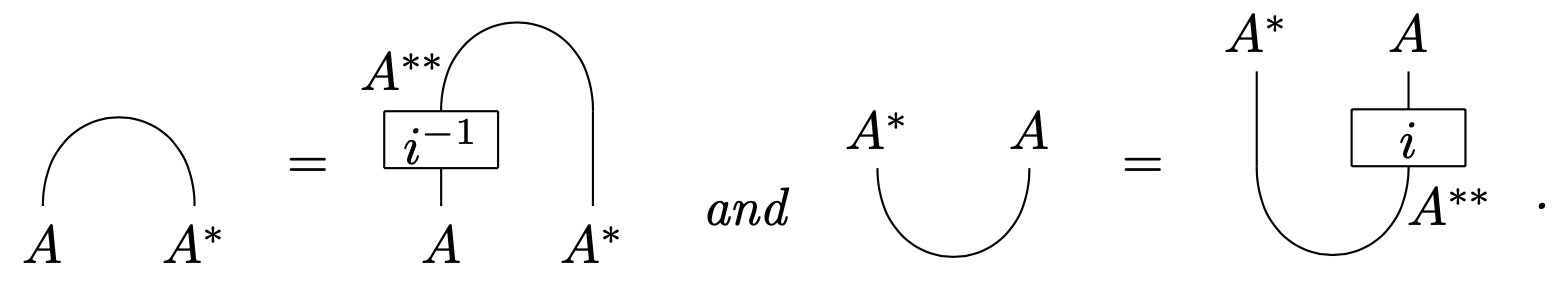The following properties are satisfied:

• These morphisms satisfy the rigidity axioms. This is, for all $A\in \mathscr{C}$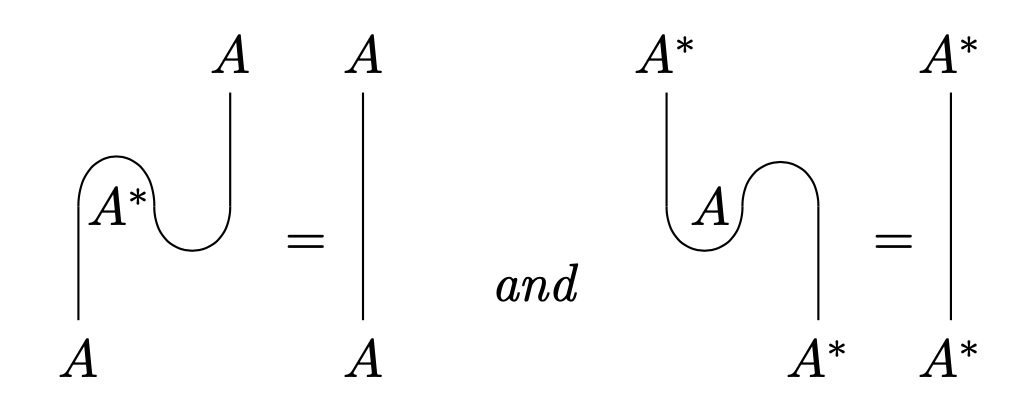• For all $A,B\in \mathscr{C}$,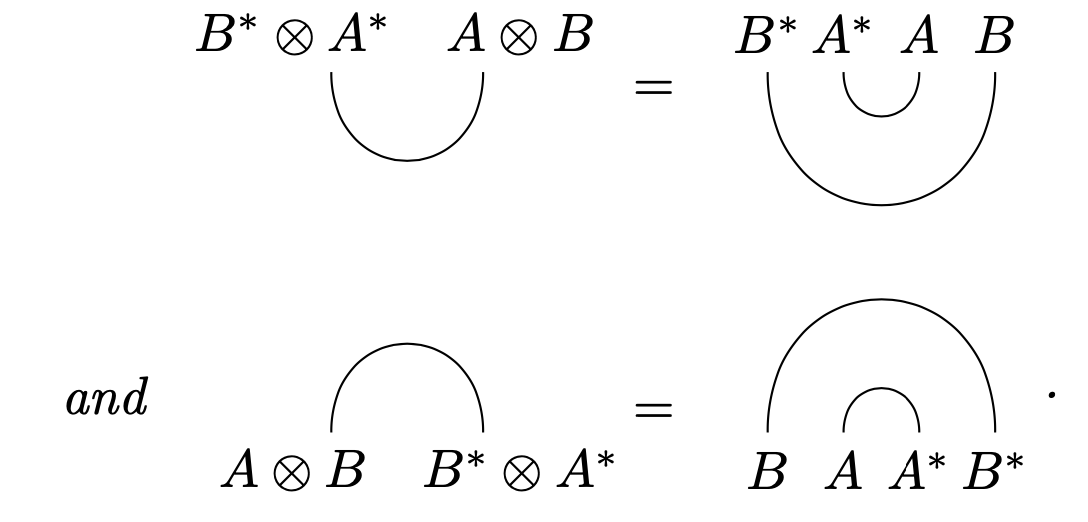• For all $A,B\in \mathscr{C}$, $f:A\to B$,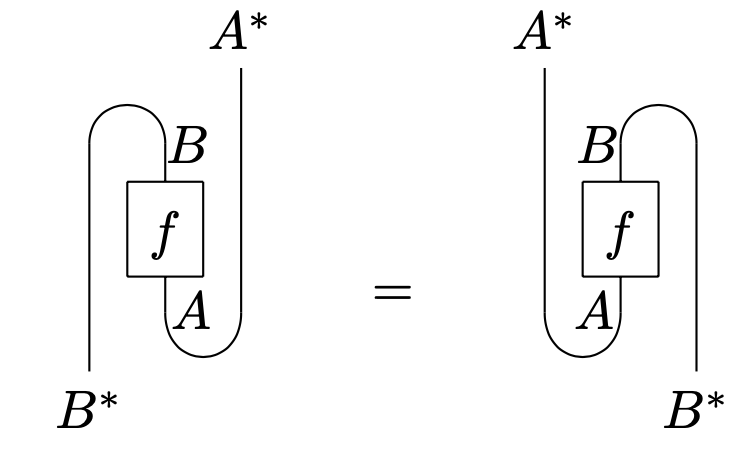Conversely, any rigid category with duals satisfying these axioms will be pivotal under the functor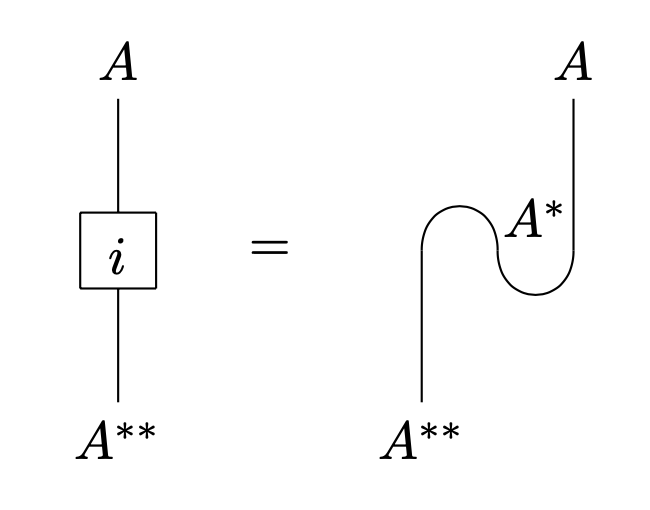This gives a bijection between pivotal categories and rigid categories with duals satisfying these axioms.

###### Proof

See Bartlett Section 5.1.

## In braided monoidal categories

It is a general principle that rigidity behaves much better in braided monoidal categories. For instance, every braided rigid category will automatically have two-sided duals (Joyal and Street, Proposition 7.2). However, there is no a-priori reason for a pivotal structure to exist. What is true is that pivotal structures can be quantified explicitly in terms of twists:

###### Proposition

[Deligne] Let $\mathscr{C}$ be a braided rigid monoidal category. Given any natural isomorphism $\theta:\id_{\mathscr{C}}\to\id_{\mathscr{C}}$ such that $\theta_{A\otimes B}=\beta_{B,A}\circ \beta_{A,B}\circ (\theta_A\otimes \theta_B)$ for all $A,B\in \mathscr{C}$, the distinguished morphisms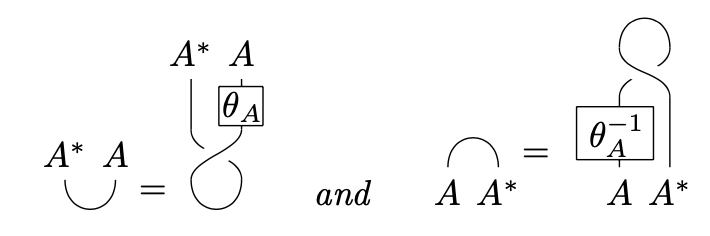give $\mathscr{C}$ the structure of a pivotal category. This map induces a canonical bijection between the set of pivotal structures on $\mathscr{C}$ and the set of natural isomorphism $\theta$ satisfying $\theta_{A\otimes B}=\beta_{B,A}\circ \beta_{A,B}\circ (\theta_A\otimes \theta_B)$ for all $A,B\in \mathscr{C}$.

###### Proof

A braided rigid monoidal category with a twist is called balanced (not to be confused with the other unrelated notion of balanced). This result can be read as saying that a braided rigid monoidal category is balanced if and only if it is pivotal. Morally, we have the following explanation:

“The lemma is remarkable because the concept of a braided autonomous category does not include any assumption relating the braided structure to the autonomous structure. Moreover, the axioms for a twist depend only on the braided structure, whereas the axioms for a pivotal structure depend only on the autonomous structure. Yet, they are equivalent if $\mathscr{C}$ is braided autonomous” - Peter Selinger

Given a pivotal structure, the twist can be recovered explicitly using graphical language as follows: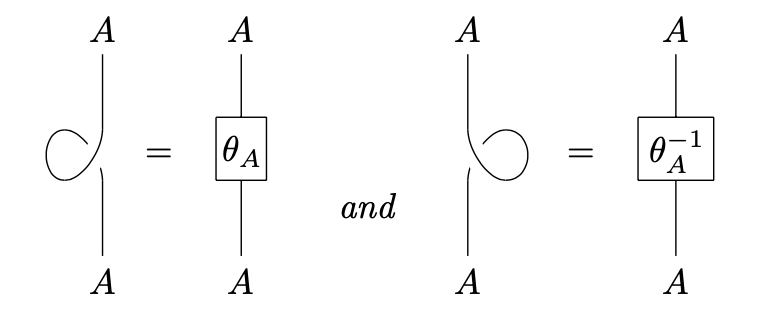The proof of this result is a simple untangling: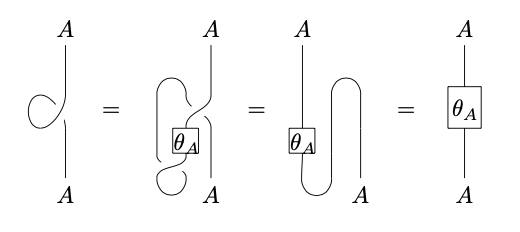## In fusion categories

In every fusion category, we automatically have that every object is isomorphic to its double dual. This is because fusion categories are semisimple, so $A\otimes A^{*}$ admitting a non-zero morphism into $1$ is the same as there being a $1$ in the simple object decomposition of $A\otimes A^{*}$, which in turn is the same as $1$ admitting a non-zero morphism into $A\otimes A^{*}$.

It is a deep conjecture of Etingof, Nikshych, and Ostrik that every fusion category is pivotal. This conjecture was proposed in their seminal work, On fusion categories. What one gets a-priori, however, is a natural isomorphism between every object and its quadruple dual, called the Radford isomorphism. This weaker isomorphism is already very powerful, and serves as an important tool in the theory of fusion categories.